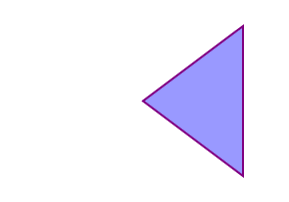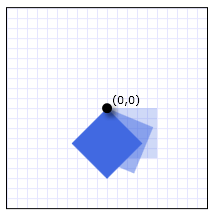# Shapes and Drawing

Microsoft Silverlight will reach end of support after October 2021. Learn more.

In Silverlight, a Shape is a type of UIElement that enables you to draw a shape to the screen. Because they are user interface (UI) elements, Shape objects can be used inside a variety of container objects such as Grid and Canvas.

This topic contains the following sections.

• Shape Objects
• Using Paths and Geometries
• Painting Shapes
• Transforming Shapes
• Related Topics

## Shape Objects

Silverlight provides a number of ready-to-use Shape objects, including Ellipse, Line, Path, Polygon, Polyline, and Rectangle. Shape objects share the following common properties:

• Stroke: Describes how the shape's outline is painted.

• StrokeThickness: Describes the thickness of the shape's outline.

• Fill: Describes how the interior of the shape is painted.

• Data properties to specify coordinates and vertices, measured in device-independent pixels.

Shape objects can be used inside Canvas objects. Canvas supports absolute positioning of its child objects through the use of the Canvas.Left and Canvas.Top attached properties.

The Line class enables you to draw a line between two points. The following example shows several ways to specify line coordinates and stroke properties.

Run this sample

``````<Canvas Height="300" Width="300">

<!-- Draws a diagonal line from (10,10) to (50,50). -->
<Line X1="10" Y1="10" X2="50" Y2="50"
Stroke="Black"
StrokeThickness="4" />

<!-- Draws a diagonal line from (10,10) to (50,50)
and moves it 100 pixels to the right. -->
<Line X1="10" Y1="10" X2="50" Y2="50"
StrokeThickness="10"
Canvas.Left="100">
<Line.Stroke>
<GradientStop Color="Red" Offset="0" />
<GradientStop Color="Blue" Offset="0.5" />
</Line.Stroke>
</Line>

<!-- Draws a horizontal line from (10,60) to (150,60). -->
<Line X1="10" Y1="60" X2="150" Y2="60"
Stroke="Black"
StrokeThickness="4"/>

</Canvas>
``````

Although the Line class does provide a Fill property, setting it has no effect because a Line has no area.

Another common shape is the Ellipse. Create an Ellipse by defining the shape's Width and Height properties, as shown in the following example. To draw a circle, specify an Ellipse whose Width and Height values are equal.

Run this sample

``````<Canvas>
<Ellipse
Fill="Yellow"
Height="100"
Width="200"
StrokeThickness="2"
Stroke="Black"/>
</Canvas>
``````

## Using Paths and Geometries

The Path class enables you to draw curves and complex shapes. These curves and shapes are described by using Geometry objects. To use a Path, you create a Geometry and use it to set the Path object's Data property. There are a variety of Geometry objects to choose from. The LineGeometry, RectangleGeometry, and EllipseGeometry classes describe relatively simple shapes. To create more complex shapes or create curves, use a PathGeometry. For information about creating PathGeometry objects, see Geometries.

### XAML Abbreviated Syntax

In XAML, you can use a special abbreviated syntax to describe a Path. In the following example, abbreviated syntax is used to draw a complex shape.

``````<Canvas>

<Path Stroke="DarkGoldenRod" StrokeThickness="3"
Data="M 100,200 C 100,25 400,350 400,175 H 280" />

</Canvas>
``````

The following illustration shows the rendered Path.

Path illustrationThe Data attribute string uses a mini-language. It begins with the move command, indicated by M, which establishes a start point for the path in the coordinate system of the Canvas. Path data parameters are case-sensitive. The capital M indicates an absolute location for the new current point. A lowercase m would indicate relative coordinates. The first segment is a cubic Bezier curve that begins at (100,200) and ends at (400,175), which is drawn by using the two control points (100,25) and (400,350). This segment is indicated by the C command in the Data attribute string. Again, the capital C indicates an absolute path; the lowercase c would indicate a relative path.

The second segment begins with an absolute horizontal line command H, which specifies a line drawn from the preceding subpath's endpoint (400,175) to a new endpoint (280,175). Because it is a horizontal line command, the value specified is an x-coordinate.

For the complete path syntax, see the Data reference and Path Markup Syntax.

## Painting Shapes

You use Brush objects to paint a shape's Stroke and Fill. In the following example, the stroke and fill of an Ellipse are specified. Note that valid input for brush properties can be either keywords or hexadecimal color values.

``````<Canvas Width="300" Height="300" Background="LightGray">
<Ellipse
Canvas.Top="50"
Canvas.Left="50"
Fill="#FFFFFF00"
Height="75"
Width="75"
StrokeThickness="5"
Stroke="#FF0000FF"/>
</Canvas>
``````

The following illustration shows the rendered Ellipse.

Rendered ellipseAlternatively, you can use property element syntax to explicitly create a SolidColorBrush object to paint the shape with a solid color.

``````<Canvas>

<!-- This polygon shape uses predefined color values for its Stroke and
Fill properties.
The SolidColorBrush Opacity property affects the fill color in
this case by making it slightly transparent (opacity of 0.4) so
that it blends with any underlying color. -->

<Polygon
Points="300,200 400,125 400,275 300,200"
Stroke="Purple"
StrokeThickness="2">
<Polygon.Fill>
<SolidColorBrush Color="Blue" Opacity="0.4"/>
</Polygon.Fill>
</Polygon>

</Canvas>
``````

The following illustration shows the rendered polygon.

Rendered polygonYou can also paint a shape's stroke or fill with gradients, images, patterns, and more. For more information, see Brushes.

## Transforming Shapes

Transform objects can be used to transform shapes (or any other UIElement) in a two-dimensional plane. The different types of transformations include rotation (RotateTransform), scale (ScaleTransform), skew (SkewTransform), and translation (TranslateTransform).

A common transform to apply to a shape is a rotation. To rotate a shape, create a RotateTransform and specify its Angle. An Angle of 45 rotates the element 45 degrees clockwise, an angle of 90 rotates the element 90 degrees clockwise, and so on. Set the CenterX and CenterY properties if you want to control the point about which the element is rotated. These property values are expressed in the coordinate space of the element that is being transformed. CenterX and CenterY have default values of 0. Finally, apply the RotateTransform to the element by setting the shape's RenderTransform property.

The following example shows a RotateTransform that rotates a Rectangle element 45 degrees about the point (0,0).

``````<Grid x:Name="LayoutRoot" Background="White">
<Rectangle Width="50" Height="50"
Fill="RoyalBlue">
<Rectangle.RenderTransform>
<RotateTransform Angle="45" />
</Rectangle.RenderTransform>
</Rectangle>
</Grid>
``````

The following illustration shows how this code is rendered.

A Rectangle rotated 45 degrees about the point (0,0)To apply multiple transforms to a shape (or to any other UI element), use a TransformGroup.